Reach Us+44-1522-440391
Sample Size Calculation for the Modified Likelihood Ratio Test in Genetic Linkage Analysis | OMICS International
Journal of Biometrics & Biostatistics

Like us on:

All submissions of the EM system will be redirected to Online Manuscript Submission System. Authors are requested to submit articles directly to Online Manuscript Submission System of respective journal.

# Sample Size Calculation for the Modified Likelihood Ratio Test in Genetic Linkage Analysis

Yuejiao Fu*, Pengfei Li and Soowoon Chung

York University and University of Waterloo, Canada

*Corresponding Author:
Yuejiao Fu
York University and University of Waterloo, Canada
Tel: 416-736-2100 ext. 33772
Fax: 416-736-5757
E-mail: [email protected]

Received date: July 01, 2014; Accepted date: August 07, 2014; Published date: August 11, 2014

Citation: Fu Y, Li P, Chung S (2014) Sample Size Calculation for the Modified Likelihood Ratio Test in Genetic Linkage Analysis. J Biom Biostat S12:002. doi:10.4172/2155-6180.S12-002

Copyright: © 2014 Fu Y, et al. This is an open-access article distributed under the terms of the Creative Commons Attribution License, which permits unrestricted use, distribution, and reproduction in any medium, provided the original author and source are are credited.

Visit for more related articles at Journal of Biometrics & Biostatistics

#### Abstract

Mixture models provide flexible means of handling heterogeneity in data. The possible latent structure suggested by mixture model analysis should be carefully examined using designed experiments. Sample size determination is an important and difficult step in design of experiments. We investigate the sample size calculation for the modified likelihood ratio test for binomial mixture models arising in genetic linkage analysis. We obtain limiting distributions for the modified likelihood ratio test under two sets of commonly used local alternatives. A simple sample-size formula is obtained and illustrated using both simulations and a genetic linkage study for schizophrenia.

#### Keywords

Contiguity theory; Genetic linkage analysis; Hypothesis testing; Local asymptotic power; Mixture models; Modified likelihood; Recombinant

#### Introduction

Mixture models provide flexible means of handling observed or unobserved heterogeneity in data. The data analysis using mixture models could unveil the possible underlying or latent structure. Welldesigned clinical trials and scientific experiments are usually needed to examine the validity of the suggested latent structure. Sample size determination is a major issue in those studies, see Chow et al.  and references therein. There is a vast literature covering sample size calculation for comparative research studies especially in medical context, for example, hypothesis testing for proportions in two groups.

Instead of considering simple designs such as a two-sample test, we consider calculating sample size for hypothesis tests in mixture model framework. More specifically we propose a formula for determining required sample size for performing a test of homogeneity. A test of homogeneity, which tests the null hypothesis of one component parametric model versus the alternative of a two-component mixture, is one of the most difficult and important problems in finite mixture models. There is some literature on power and sample size calculations for tests of homogeneity in finite mixture models. Hall and Stewart  provided theoretical analysis of power in a two-component normal mixture model and addressed the irregular feature of the problem. Recently, Chen et al.  addressed the issue of sample size calculation for tests of homogeneity using the EM-test and C(α) test. Instead of a general homogeneity test, we consider a special binomial mixture model arising in genetic linkage analysis. This particular binomial mixture model in pedigree studies has been studied in Lemdani and Pons , Liang and Rathouz , Fu et al . showed that the modified likelihood ratio test (MLRT) which was proposed by Chen  and Chen et al.  has better power for detecting the aforementioned binomial mixture alternative than other methods discussed in their paper. Since sample size calculation is test-specific, for the homogeneity test of the special binomial mixture, we choose the MLRT as the basis for the sample size determination. Following Chen et al. , we investigate the power properties of the MLRT under two sets of commonly used local alternatives. A simple sample size formula is obtained and illustrated by both simulations and a genetic linkage study for schizophrenia.

The rest of the article is as follows. Section 2 presents the problem set up and gives the asymptotic distribution of the MLRT and samplesize formula for two local alternatives. Section 3 presents a real data example in genetic linkage study and simulation results are given in Section 4. The proof of the theorem is given in the Appendix.

#### Main Results

The particular binomial mixture model in pedigree studies we consider is a two-component binomial mixture with one component distribution completely known. This model is commonly used to model the recombinant data in pedigree studies and known as phase known model. See Liang and Rathouz  and Fu et al.  for more details. Suppose we have a random sample X1,…,Xn drawing from the following binomial mixture model

(1−γ)Bin(m,0.5) + γBin(m,θ),

where γ is the mixing proportion and θ ∈ [0,0.5] is the component parameter with a specified range. Our interest is to test homogeneity with the null hypothesis specified as

H0 : γ (θ −0.5)=0.

Note that there are two unusual features of the homogeneity test: (1) the null hypothesis lies on the boundary of the parameter space, and (2) the parameters γ and θ are not identifiable under the null model. The log-likelihood function of (γ,θ) is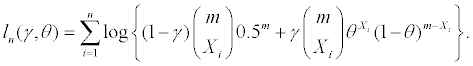The modified log-likelihood function is defined as

pln(γ,θ) = ln(γ,θ) + Clog(γ)

with C>0. In this paper, we choose C=1 as suggested in Fu et al. . The MLRT statistic is defined as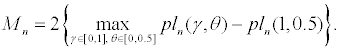The limiting distribution of Mn is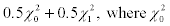denotes a degenerate distribution with all its mass at zero. Given a significance level α<0.5, the MLRT rejects H0 when Mn > z2α , where zα is the αth upper quantile of standard normal distribution.

The key step of sample size determination is to find the distributions of the test statistics under alternative hypotheses. However, such distributions are usually not available. In the context of homogeneity test, along the same line of Chen et al. , we consider power and sample size calculations under local alternative models. Among many possible deviations from the null model, we choose the following local alternatives which are contiguous to the null distribution see Le Cam and Yang :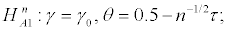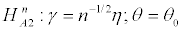(1)

where γ0 and θ0 are constants within the parameter space. For testing homogeneity in finite mixture models, we usually encounter two types of loss of identifiability, which lead to the two specified local alternatives.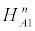refers to the situation where two-component distributions are close to each other, and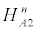refers to the situation where one mixing proportion is close to 0. In pedigree studies,suggests even for the families with linkage the linkage is weak; whilesuggests that there hardly exist any families with disease locus linked with the marker under consideration.

From Le Cam's contiguity theory, the limiting distribution of the MLRT statistic Mn under two specified local alternativesorcan be determined. The results are given in the following theorem and the proof is in the Appendix.

Theorem 1. Let δ=n1/2γ(0.5−θ). Underorwe have as n→∞,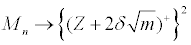in distribution, where Z denotes a standard normal random variable.

Note that under the two specified local alternatives, δ does not depend on n. It is equal to γ0τ underand η(0.5−θ0) under. We use the above asymptotic distribution of Mn under the two specified local alternativesoras the basis for power and sample size calculations. For a given alternative model (1−γ)Bin(m,0.5) + γBin(m,θ), the local power of the MLRT can be approximated by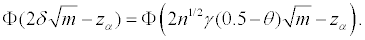(2)

Note that the three basic components of sample size calculation are significance level α, target power 1−β and a potential alternative model. For the two sequences of local alternative modelorif the target power is 1−β at a significance level α, the required sample size approximately satisfies the following equation: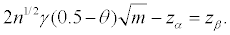In other words, the minimum sample size requirement is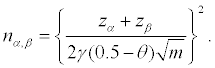The validity of the sample size formula is examined using a real data example and computer simulations which are given in the next two sections.

#### Application

We applied the developed theory to a genetic linkage study for schizophrenia conducted at the Johns Hopkins School of Medicine. The details of the study design and data collection can be found in Pulver et al.  and Liang and Rathouz . This study included 486 individuals from 54 families with at least two affected relatives. Here "affected" refers to someone who was diagnosed with either schizophrenia or schizoaffective disorder based on the DSM-III-R criteria. Based on previous studies, one is particularly interested in Marker D22S941 on chromosome 22. However, it is well known that schizophrenia is prone to heterogeneity. Research showed that the following two-component binomial mixture

0.6Bin(9,0.5) + 0.4Bin(9,0.06)

may fit the data well. Suppose our interest is to validate above mixture structure at the 0.5% level, which is typical in linkage studies, with at least 80% power. The approximate sample size is n0.005,0.2 ≈10. We also used computer simulations to check: (1) whether the limiting distribution provides reasonable approximation to the finite sample distribution under the calculated sample size; (2) whether the MLRT statistic has the desired power to detect the heterogeneity. In the simulations, we set C=1 as recommended by Fu et al. . The simulated type I error is 0.4%, and the power of Mn is 87% based on 50,000 repetitions.

Similarly, we consider the situation where the significance level is 1%, and target power is 80%. The approximate sample size is n0.01,0.2 ≈ 9.The simulated type I error and power of Mn are around 1.4% and 86%, respectively.

#### Simulation Study

We further examined the performance of the sample size calculation formula under other settings. We considered eight alternative models which are determined by the various combinations of γ=(0.05,0.3), θ=(0.05,0.3), and m=(4,8). We considered two significance levels 0.5% and 1%, with the same desired power 80%. For each alternative model, we calculated the required sample size, the simulated type I error rate, and power of Mn with C=1 based on 50,000 repetitions. The results were summarized in Tables 1 and 2. From the tables we can see that the proposed sample size formula reliably achieves the desired power under different alternative models.

Table 1: Simulated type I error and power of Mn with C=1 under the null model and under the given alternative model (1−)Bin(m,0.5) + γBin(m,θ). The significance level is 0.5%.

Table 2: Simulated type I error and power of Mn with C=1 under the null model and under the given alternative model (1−)Bin(m,0.5) + γBin(m,θ). The significance level is 1%.

#### Acknowledgements

The research was partially supported by grants from the Natural Sciences and Engineering Research Council of Canada. The authors would like to thank the editor, and two referees for their valuable suggestions and comments.

#### References

Select your language of interest to view the total content in your interested language

### Article Usage

• Total views: 12646
• [From(publication date):
specialissue-2015 - Sep 21, 2019]
• Breakdown by view type
• HTML page views : 8821

## Post your commentCan't read the image? click here to refresh
###### Peer Reviewed Journals

Make the best use of Scientific Research and information from our 700 + peer reviewed, Open Access Journals

International Conferences 2019-20

Meet Inspiring Speakers and Experts at our 3000+ Global Annual Meetings

Top